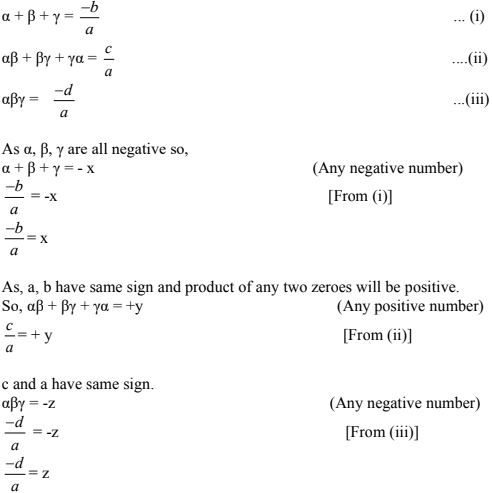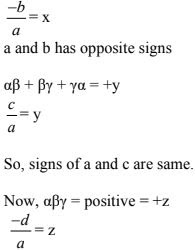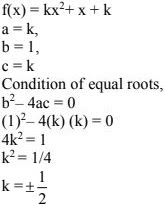# Chapter 2 Polynomials NCERT Exemplar Solutions Exercise 2.2 Class 10 MathsChapter Name NCERT Maths Exemplar Solutions for Chapter 2 Polynomials Exercise 2.2 Book Name NCERT Exemplar for Class 10 Maths Other Exercises Exercise 2.1Exercise 2.3Exercise 2.4 Related Study NCERT Solutions for Class 10 Maths

### Exercise 2.2 Solutions

1. Answer the following and justify:
(i) Can x2 – 1 be the quotient on division of x6 + 2x3 + x - 1 by a polynomial in x of degree 5 ?
(ii) What will the quotient and remainder be on division of ax2 + bx + c by px3 + qx2 + rx + s, p ≠ 0 ?
(iii) If on division of a polynomial p(x) by a polynomial g(x), the quotient is zero, what is the relation between the degrees of p (x) and g (x)?
(iv) If on division of a non-zero polynomial p (x) by a polynomial g (x), the remainder is zero, what is the relation between the degrees of p (x) and g (x)?
(v) Can the quadratic polynomial x2 + kx + k have equal zeroes for some odd integer k ? 1 ?

Solution
(i) No, x2 - 1 cannot be the quotient  on division of x6 + 2x3 + x - 1 by a polynomial in x of  degree 5.
Explanation :
When a polynomial with degree 6 is divided by degree 5 polynomial, the quotient will be of degree 1.
Assuming that (x2 - 1) divides the degree 6 polynomial and the quotient obtained is degree 5 polynomial.
As a = bq + r, so,
(Degree 6 polynomial) = (x2 - 1) (degree 5 polynomial) + r(x)
= (degree 7 polynomial) + r(x)
[As, (x2 term × x5 term = x7 term)]
= (degree 7 polynomial)
Hence, x2 - 1 cannot be the quotient on division of x6 + 2x3 + x - 1 by a polynomial in x of degree 5.

(ii) Degree of the polynomial px3 + qx2 + rx + s = 3
Degree of the polynomial ax2 + bx + c = 2
Here, Degree of px3 + qx2 + rx + s is greater than degree of the ax2 + bx + c
Hence, the quotient would be zero,
Therefore, the remainder would be the dividend = ax2 + bx + c.

(iii) We have, p(x) = g(x) × q(x) + r(x)
As given in the question,
q(x) = 0
When q(x) = 0,
r(x) is also = 0
Here, when we divide p(x) by g(x),
Then, p(x) should be = 0
Therefore, the relation between the degrees of p(x) and g(x) is the degree p(x) < degree g(x).

(iv) To divide p(x) by g(x)
We have,
Degree of p(x) > degree of g(x)
or
Degree of p(x) = degree of g(x)
Hence, the relation between the degrees of p(x) and g(x) is degree of p(x) > degree of g(x)

(v) A Quadratic Equation has equal roots when :
b2 - 4ac = 0
Given,
x2 + kx + k = 0
a = 1,
b = k,
x = k
Putting values in the equation we get,
k2 - 4(1)(k) = 0
⇒ k3 - 4k = 0
⇒ k(k - 4) = 0
⇒ k = 0,
⇒ k = 4
Here, it is given that k is greater than I, So, the value of k is 4 if the equation has common roots.
If k = 4, then the equation (x2 + kx + k) will have equal roots.

(i) If the zeroes of a quadratic polynomial ax2 + bx + c are both positive, then a, b and c all have the same sign.
(ii) If the graph of a polynomial intersects the x - axis at only one point, it cannot be a quadratic polynomial.
(iii) If the graph of a polynomial intersects the x - axis at exactly two points, it need not be a quadratic polynomial.
(iv) If two of the zeroes of a cubic polynomial are zero, then it does not have linear and constant terms.
(v) If all the zeroes of a cubic polynomial are negative, then all the coefficients and the constant term of the polynomial have the same sign.
(vi) If all three zeroes of a cubic polynomial x3 + ax2 - bx + c are positive, then at least one of a, b and c is non - negative.
(vii) The only value of k for which the quadratic polynomial kx2 + x + k has equal zeros is 1/2.

Solution

(i) False
Taking α and β as the roots of he quadratic polynomial. If α and β are positive then α + β = -b/a. For making sum of roots positive either b or a must be negative.

(ii) False
The statement is false, because when two zeroes of a quadratic polynomial are equal, then two intersecting points coincide to become one point.

(iii) True
If a polynomial of degree more than two has two zeroes and other zeroes are not real or are imaginary, then graph of the polynomial will intersect at two points on x-axis.

(iv) True
Taking,
β = 0,
γ = 0
f(x) = (x - α)(x - β)(x - γ)
= (x - α) x . x
f(x) = x3 - ax2
So, it has no linear (coefficient of x) and constant terms.

(v) True
α, β and γ are all negative for cubic polynomial ax3 + bx2 + cx + d.Here, d and a will have same sign.
So, sign of b, c, d are same as of a.
Signs of a, b, c, d will be same either positive or negative.

(vi) False: As all zeroes of cubic polynomial are positive
let f(x) = x3 +  ax2 - bx + c
α + β + γ = positive, (say + x)a and d have opposite signs.
Therefore, we can conclude that,
From (i) if a is positive, then b is negative.
From (ii) if a is positive, then c is also positive.
From (iii) if a is positive, then d is negative.
So, if zeroes α, β, γ of cubic polynomial are positive then out of a, b, c at least one is negative.

(vii) FalseSo, the values of k are ± 1/2 so that the given equation has equals roots.